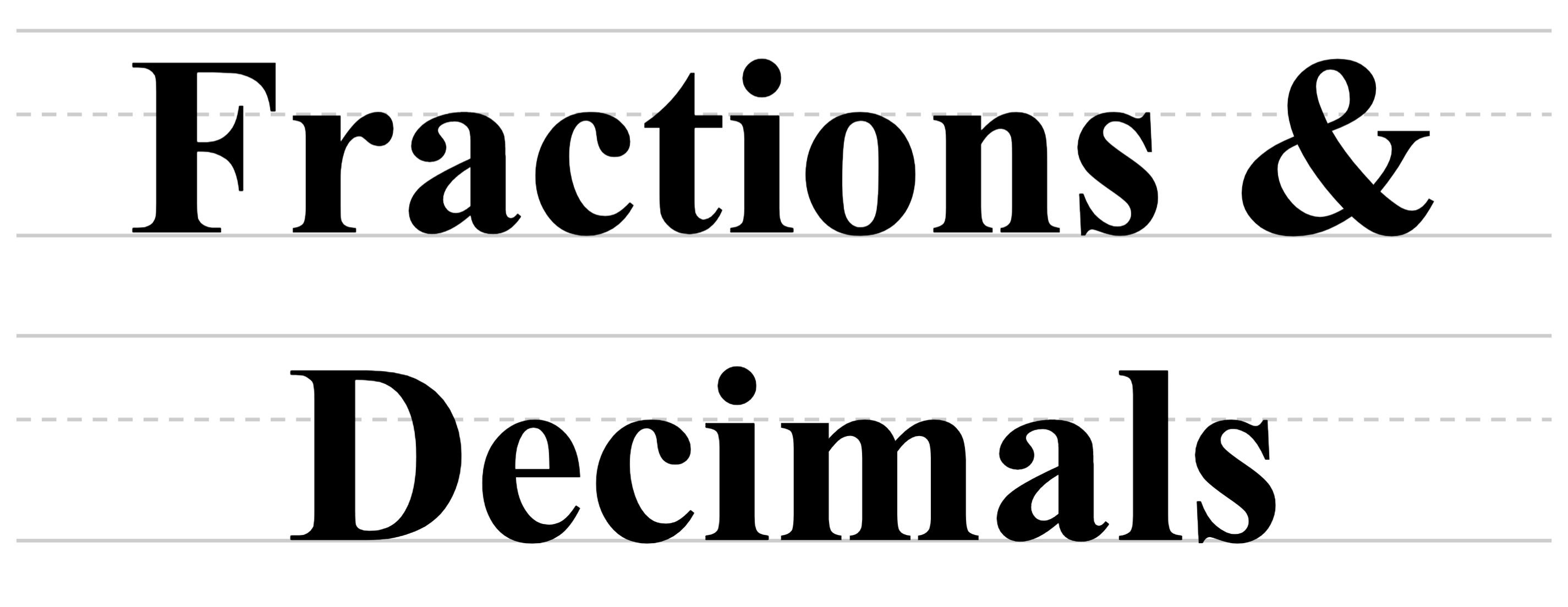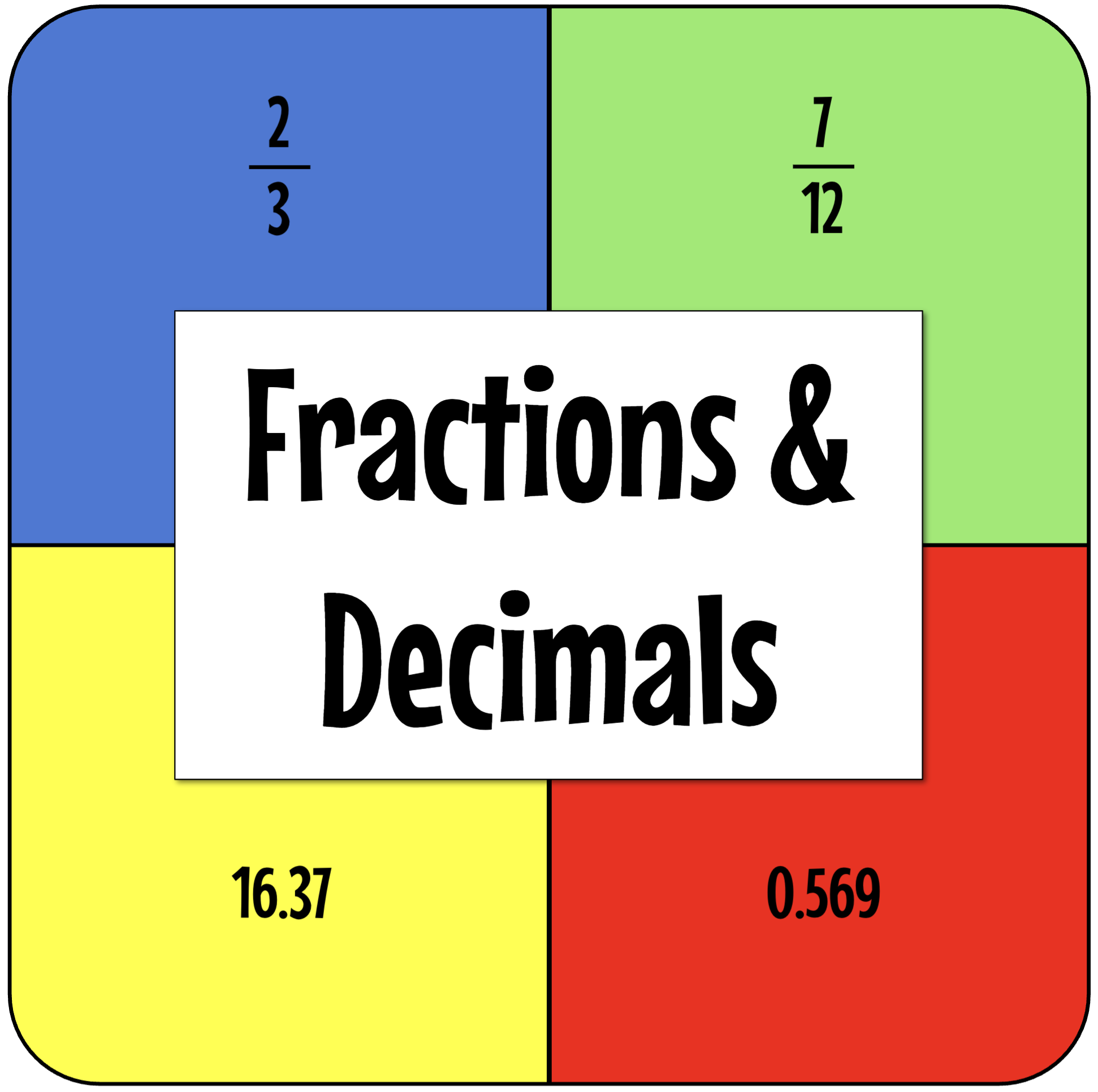Back to Mr. Anker Tests, Grade 4There are many sections below, including identifying, naming,
adding, subtracting, multiplying and dividing fractions and decimals.
There are also sections on improper fractions and mixed numbers,
along with simplifying fractions and others.

Teachers & Parents: It is recommended that students master each activity before moving on to the next.

Naming Fractions (for review, if necessary)
 Fractions 1Simple fractions with clear illustrations, no simplification is used here.(20 Questions) Fractions 2More complex fractions with clear illustrations, no simplification is used.(20 Questions) Fractions 3Even more complex fractions with clear illustrations, still no simplification is used.(20 Questions) Fractions 4Complex fractions with clear illustrations, simplification is required.(20 Questions)

 Adding Fractions 1Adding fractions with clear illustrations, simplification is used on the last question only. Adding Fractions 2Adding fractions with clear illustrations, simplification is used on the last question only. Adding Fractions 3Adding fractions without illustrations, simplification is not used. Adding Fractions 4Add fractions without illustrations. Requires finding the Least Common Denominator (LCD).
 Adding Fractions 5Add fractions without illustrations. Some questions require simplification of both fraction addends and the final answer. Includes a tutorial on finding the Least Common Denominator(LCD).

Subtracting Fractions
 Subtracting Fractions 1Subtract fractions without illustrations, though all with like denominators. Simplification is not used. Subtracting Fractions 2About the same level as Subtraction 1, all questions with like denominators. Simplification is not used. Subtracting Fractions 3All questions with unlike denominators. Simplification of difference (answer) not required. Subtracting Fractions 4About the same level as Subtraction 3. Simplification of difference (answer) not required.
 Subtracting Fractions 5Similar to Subtracting Fractions 4. All questions require simplification of either the fraction subtrahend and minuend, as well as the final answer. Subtracting Fractions 6Similar to Subtracting Fractions 5. All questions require simplification of either the fraction subtrahend and minuend, as well as the final answer.

Multiplying Fractions
 Multiplying Fractions 1Multiply simple fractions. Simplification not needed for this activity, though numbers will be larger. Multiplying Fractions 2Multiply simple fractions. Answers must be simplified. Simplification model included. Multiplying Fractions 3Multiply simple fractions, some of which will require simplification. Multiplying Fractions 4Same skills as Multiplying Fractions 3.
 Multiplying Fractions 5Multiply simple fractions using cancelling method and simplification. Multiplying Fractions 6Multiply simple fractions. Cancellation & simplified needed. Simplification model included. Multiplying Fractions 7Multiply fractions with larger numbers. Cancellation & Simplification needed.

Dividing Fractions
 Dividing Fractions 1Divide simple fractions. Answers must be entered in simplest form. Dividing Fractions 2Divide simple fractions. Answers must be entered in simplest form. Dividing Fractions 3Divide a fraction by a whole number. Dividing Fractions 4Divide a fraction by a whole number (larger values are used).
 Dividing Fractions 5Divide a whole number by a fraction.(10 Questions) Dividing Fractions 6Divide a whole number by a fraction (larger values are used).(10 Questions) Dividing Fractions 7Divide with fractions and whole numbers.(10 Questions)

Simplifying Fractions
 Simplifying Fractions 1Simplify easy fractions. Hints are available on all exercises. Simplifying Fractions 2Simplify easy fractions. Hints are available on a few exercises. Simplifying Fractions 3Simplify easy fractions. Hints are available on a few exercises. Simplifying Fractions 4Simplify easy fractions. Hints are available on a few exercises.
 Simplifying Fractions 5Simplify easy fractions. Simplifying Fractions 6Simplify easy fractions. Simplifying Fractions 7Simplify easy fractions. Simplifying Fractions 8Solving simple addition & subtraction of fractions, then simplify.

Improper Fractions & Mixed Numbers
 Improper Fractions 1Convert a mixed number to an improper fraction. Improper Fractions 2Convert a mixed number to an improper fraction.(larger values are used) Improper Fractions 3Convert a mixed number to an improper fraction.(larger values are used) Improper Fractions 4Convert a mixed number to an improper fraction.(larger values are used)
 Mixed Numbers 1Convert an improper fraction to a mixed number. Mixed Numbers 2Convert an improper fraction to a mixed number (larger values are used). Mixed Numbers 3Convert an improper fraction to a mixed number (larger values are used). Mixed Numbers 4Convert an improper fraction to a mixed number (larger values are used).
 Mixed Numbers 5Convert an improper fraction to a mixed number; compare mixed numbers with improper fractions. Mixed Numbers 6Convert an improper fraction to a mixed number; compare mixed numbers with improper fractions. Mixed Numbers 7Convert an improper fraction to a mixed number; compare mixed numbers with improper fractions. Mixed Numbers 8Convert an improper fraction to a mixed number; compare mixed numbers with improper fractions.

Fractions on a Number Line & Equivalent Fractions
 Fractions on a Number Line 1Identify the location of the fraction on the number line.(20 Questions) Fractions on a Number Line 2Identify the location of the fraction on the number line.(20 Questions) Equivalent Fractions 1Identify equivalent fractions.(10 Questions) Equivalent Fractions 2Identify equivalent fractions.(10 Questions)

Fractions of Whole Numbers
 Fractions of a Whole Number 1Find the fraction of a whole number. Small values. Fractions of a Whole Number 2Find the fraction of a whole number. Small values. Fractions of a Whole Number 3Identify equivalent fractions.Slightly larger values. Fractions of a Whole Number 4Identify equivalent fractions.Slightly larger values.
 Fractions of a Whole Number 5Find the fraction of a whole number.Larger values. Fractions of a Whole Number 6Find the fraction of a whole number.Small values. Half of Whole Numbers 1Using mental math, find half of a whole number.(20 questions) Half of Whole Numbers 2Using mental math, find half of larger whole numbers.(20 questions)

Decimals on a Number Line and Fractions & Decimals
 Decimals on a Number Line 1Not yet ready. Decimals on a Number Line 2Not yet ready. Fractions & Decimals 1Understand that fractions & decimals are two different ways to represent the same value. Fractions & Decimals 2Understand that fractions & decimals are two different ways to represent the same value.

Comparing Decimals
 Comparing Decimals 1Compare decimals to the hundredths place. Comparing Decimals 2Compare decimals to the thousandths place. Comparing Decimals 3Compare decimals to the ten thousandths place. Comparing Decimals 4Compare decimals to the hundred thousandths place.

Rounding Decimals
 Rounding Decimals 1Round values under 10 to the nearest whole number. Rounding Decimals 2Round values under 100 to the nearest whole number. Rounding Decimals 3Round values under 100 to the nearest 10th. Rounding Decimals 4Round values under 100 to the nearest 100th.
 Rounding Decimals 5Round values with many decimal places to the nearest whole number. Rounding Decimals 6Round values with many decimal places to the nearest whole number, 10th and 100th. Rounding Decimals 7Round values with many decimal places to the nearest whole number, 10th and 100th. Rounding Decimals 8Round values with many decimal places to the nearest whole number, 10th and 100th.

Writing Decimals
 Writing Decimals 10thsWrite decimal values with words and numbers to the tenths place. Writing Decimals 100thsWrite decimal values with words and numbers to the hundredths place. Writing Decimals 1000thsWrite decimal values with words and numbers to the thousandth place. Writing Decimals MixedWrite decimal values with words and numbers to the tenths, hundredths, and thousandths place.